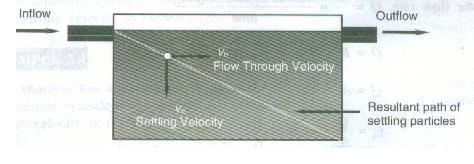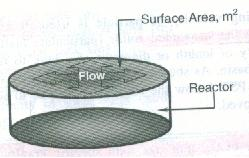Feb 26, 2016

Feb 26, 2016

Feb 26, 2016

# Methods of Sewage Waste disposal

Methods of sewage disposal are of two types:

1. Dry or conservatory system
2. Water carriage system (99.9)

## Detention Time

Detention Time (Hydraulic Retention Time, HRT) is the length of time a particle or a unit volume of water/wastewater remains in a reactor/basin/tank.

Also See: Types of Hospital Waste

Mathematically;

HRT, tD = (Volume/Discharge) ------- days/hours/sec (units)

Flow Through Velocity or Horizontal Velocity and Settling VelocitySettling path of a solids particle in a tank

Surface Loading Rate or Overflow Rate is the volume of water/wastewater applied per unit surface area of the treatment basin and is normally expressed in units of m3/m2-d or gal/ft2-d.

Mathematically;

SLR = (Flow, Q/Area, As) {Numerically; SLR = HRT}Weir Loading Rate or Weir Overflow Rate is the quantity of wastewater flowing over a unit weir length of the tank in a unit time. It is expressed in units of m3/m-d or gal/ft-d.

Mathematically;

WLR = (Flow, Q/Total Length of Weir, L)

PROBLEM: A treatment unit is 1.5 ft wide, 20 ft long and has a depth of 2.0 ft. if the wastewater flow rate through the tank is 0.5 ft3/sec. Calculate; detention time, overflow rate and weir loading rate.

Organic Loading is the total quantity of organic matter in terms of BOD or COD that is applied per day over the unit surface area of per unit volume of the treatment basin or tank.

Mathematically;

OLR = (applied kg of BOD per day)/(volume of the basin)

It is expressed as kg-BOD/ m3-d or kg-COD/ m3-d

PROBLEM: A treatment basin has a diameter of 20 ft and a depth of 2.5 ft. Calculate the organic loading rate for an effluent of 10.0 MGD having 220 mg/L of BOD.

Food-Microorganisms (F/M) Ratio is the relationship between the available food (F) and the microorganism (M) present in a biological treatment system.

F/M = [(Q*So)/(V*X)] ----------- it is expressed in unit of sec-1 or d-1 etc.
Since, HRT tD = V/Q, therefore, F/M = [So/(tD*X)]

PROBLEM: If the wastewater with BOD concentration of 200 mg/L is treated in a tank having 5 hours HRT and containing 4000 mg/L MLVSS, calculate its F/M ratio.

Mean Cell Residence Time or Solid Retention Time or Sludge Age; is the average time in days for which biomass are retained in the biological reactors.